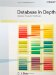# Free and Bound Variables

 I said earlier that another term for what I've been calling parameters is free variables. Quantifying over a free variable converts it into a bound variable. For example, consider again the 2-place predicate q from the previous section: ` x is taller than y ` The parameters x and y here are free variables. If we now "quantify existentially" over x, we obtain: ` EXISTS x ( x is taller than y ) ` Here, y is still free, but x is now bound. And in the "fully quantified" form: ` EXISTS x EXISTS y ( x is taller than y ) ` x and y are both bound, and there are no free variables at all (the predicate has degenerated to a proposition). Now, we already know that free variables correspond to parameters, in conventional programming terms. Bound variables, by contrast, don't have an exact counterpart in conventional programming terms at all; instead, they serve as a kind of dummy they serve only to link the predicate inside the parentheses to the quantifier outside. For example, consider the simple predicate (actually a proposition): ` EXISTS x ( x > 3 ) ` This proposition merely asserts that there exists some integer greater than three. (I'm assuming that x here is constrained to "range over" the set of integers. Again, this is a point I'll come back to in the section "Database Constraints.") Note, therefore, that the meaning of the proposition would remain totally unchanged if the two x's were both replaced by some other variable y. In other words, the proposition: ` EXISTS y ( y > 3 ) ` is semantically identical to the one just shown. Now consider the predicate: ` EXISTS x ( x > 3 ) AND x < 0 ` Here there are three x's, but they don't all denote the same thing. The first two are bound and can be replaced by, say, y without changing the overall meaning; but the third is free and cannot be replaced with impunity. Thus, of the following two predicates, the first is equivalent to the one just shown and the second is not: ` EXISTS y ( y > 3 ) AND x < 0 EXISTS y ( y > 3 ) AND y < 0 ` To close this section, I remark that we can now (re)define a proposition to be a predicate in which all of the variables are bound.Database in Depth: Relational Theory for Practitioners
ISBN: 0596100124
EAN: 2147483647
Year: 2006
Pages: 127
Authors: C.J. Date

Similar book on Amazon